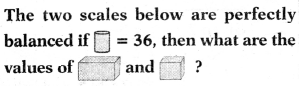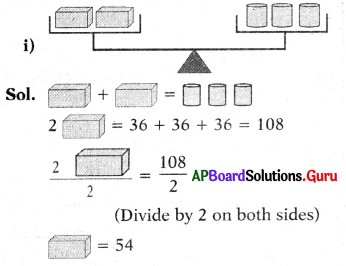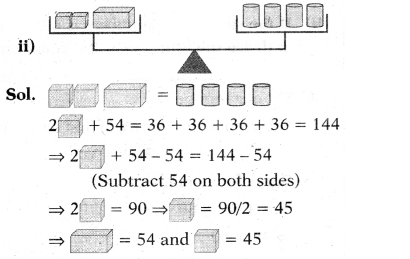# AP Board 7th Class Maths Solutions Chapter 3 Simple Equations Ex 3.4

SCERT AP 7th Class Maths Solutions Pdf Chapter 3 Simple Equations Ex 3.4 Textbook Exercise Questions and Answers.

## AP State Syllabus 7th Class Maths Solutions 3rd Lesson Simple Equations Exercise 3.4

Question 1.
Find the height of the statue from the adjacent diagram.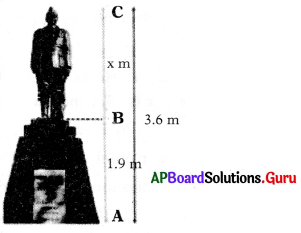From the given diagram
Height of the statue (BC) = x m
Height of the Pedastal (AB) = 1.9 m
Total Height (AC) = 3.6 m
BC + AB = 3.6 m
⇒ x + 1.9 m = 3.6 m
⇒ x + 1.9 – 1.9 = 3.6 – 1.9 (Subtract 1.9 on both sides)
⇒ x = 1.7 m
∴ Height of the statue = 1.7 mQuestion 2.
The sum of twice a number and 4 is 80, find the number.
Let the number be x.
Twice the number = 2x
Sum of twice a number and 4 = 80
2x + 4 = 80
⇒ 2x + 4 – 4 = 80 – 4
(Subtract 4 on both sides)
⇒ 2x = 76
⇒ $$\frac{2 x}{2}$$ = $$\frac{76}{2}$$ (Divide by 2 on both sides)
⇒ x = 38
∴ Number = 38

Question 3.
The difference between a number and one-fourth of itself is 24, find the number.
Let the number be a.
One-fourth of a number = $$\frac{1}{4}$$ of a = $$\frac{a}{4}$$
Difference of number and one-fourth of itself = 24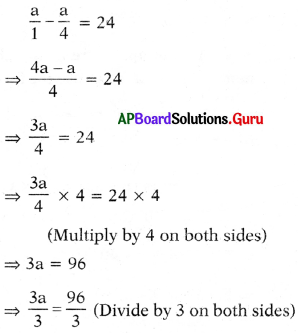⇒ a = 32
∴ The number a = 32

Question 4.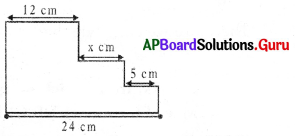Find the value of x from the adjacent diagram.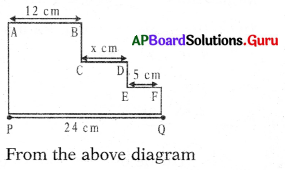From the above diagram
AB = 12 cm; CD = x cm
EF = 5 cm; PQ = 24 cm
We know,
AB + CD + EF = PQ
⇒ 12 + x + 5 = 24
⇒ x + 17 = 24
⇒ x + 17 – 17 = 24 – 17
(Subtract 17 on both sides)
⇒ x = 7 cmQuestion 5.
To convert temperature from Fahrenheit to Centigrade, we use the formula (F – 32) = $$\frac{9}{5}$$ × C. If C = – 40° C, then find F.
Given formula (F – 32) = $$\frac{9}{5}$$ × C and C = – 40° C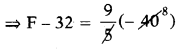⇒ F – 32 = 9(- 8)
⇒ F – 32 = – 72
⇒ F – 32 + 32 = – 72 + 32 (Add 32 on both sides)
⇒ F = – 40° F
Therefore we conclude that
– 40° C = – 40° F.

Question 6.
Rahim has ₹x, from which he spends ₹6. If twice of the money left with him is ₹86, then find x.
Rahim has money = ₹x
Money after spends ₹6 = x – 6
Twice the money left with Rahim
= 2(x – 6) = ₹86
⇒ – 2(x – 6) = 86
⇒ $$\frac{2(x-6)}{2}$$ = $$\frac{86}{2}$$ (Divide by 2 on both sides)
⇒ x – 6 = 43
⇒ x – 6 + 6 = 43 + 6 (Add 6 on both sides)
⇒ x = 49
Therefore money at Rahim = ₹49.

Question 7.
The difference between two numbers is 7. Six times the smaller plus the larger is 77. Find the numbers.
Let the smaller number is x.
Larger number = x + 7
Six times the smaller = 6.x
Six times smaller plus the larger = 77
⇒ 6x + x + 7 = 77
⇒ 7x + 7 = 77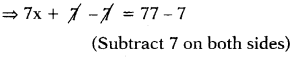⇒ 7x = 70
⇒ $$\frac{7 \mathrm{x}}{7}$$ = $$\frac{70}{7}$$ (Divide by 7 on both sides) ⇒x = 10
∴ Smaller number x = 10
Larger number = x + 7 = 10 + 7 = 17
∴ The numbers are 10 and 17.Question 8.
The sum of three consecutive even numbers is 54. Find the numbers.
Let the three consecutive even numbers are 2x, 2(x +1), 2(x + 2).
Sum of three consecutive even numbers = 54
⇒ 2x + 2(x + 1) + 2(x + 2) = 54
⇒ 2x + 2x + 2 + 2x + 4 = 54
⇒ 6x + 6 = 54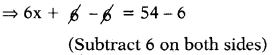⇒ 6x = 48
⇒ $$\frac{6 x}{6}$$ = $$\frac{48}{6}$$ (Divide by 6 on both sides)
⇒ x = 8
Numbers are, 2x, 2(x + 1), 2(x + 2)
2(8), 2(8 + 1), 2(8 + 2)
∴ The numbers are 16, 18, 20.

Question 9.
In a class of 48 students, the number of girls is one third the number of boys. Find the number of girls and boys in the class.
Let the number of boys = x
The number of girls = $$\frac{1}{3}$$ of boys
= $$\frac{1}{3}$$ of x = $$\frac{x}{3}$$
Boys + Girls = Total students
⇒ $$\frac{x}{1}+\frac{x}{3}$$ = 48
⇒ $$\frac{3 x+x}{3}$$ = 48
⇒ $$\frac{4 x}{3}$$ = 48
⇒ $$\frac{4 x}{3}$$ × 3 = 48 × 3
(Multiply by 3 on both sides)
⇒ 4x = 144
⇒ $$\frac{4 x}{4}$$ = $$\frac{144}{4}$$ (Divide by 4 on both sides)
⇒ x = 36
∴ Number of boys = 36
Number of girls = $$\frac{x}{3}$$ = $$\frac{36}{3}$$ = 12
Boys = 36 and girls = 12Question 10.
The present ages of Mary and Joseph are in the ratio 5 : 3. After 3 years sum of their ages will be 38. Find their present ages.
Ratio of present ages of Mary and Joseph = 5:3 = 5x : 3x
After 3 years their ages
= 5x + 3 : 3x + 3
Sum of their ages = 38
⇒ 5x + 3 + 3x + 3 = 38
⇒ 8x + 6 = 38
⇒ 8x + 6 – 6 = 38 – 6 (Subtract 6 on both sides)
⇒ 8x = 32
⇒ $$\frac{8 x}{8}$$ = $$\frac{32}{8}$$
⇒ x = 4
⇒ Ratio of present ages = 5x : 3x
= 5(4) : 3(4) = 20 : 12
Present ages of Mary and Joseph are 20 years and 12 years.

Question 11.
A sum of ₹500 is in the form of notes of denominations of ₹5 and ₹10. If the total number of notes is 90, then find the number of notes of each type.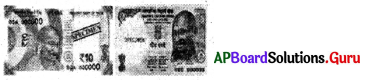Let, number of f5 notes = x
Given sum of number of ₹5 notes and ₹10 notes is 90.
So, number of ₹10 notes = 90 – x
Sum of ₹5 notes = ₹5 × x = 5x
Sum of ₹ 10 notes = ₹ 10 × (90 – x)
= 900 – 10x
⇒ 5x + 900 – 10x = 500
⇒ – 5x + 900 = 500
⇒ – 5x + 900 – 900 = 500 – 900 (Subtract 900 on both sides)
⇒ – 5x = – 400
⇒ $$\frac{-5 x}{-5}$$ = $$\frac{-400}{-5}$$
(Divide by – 5 on both sides)
⇒ x = 80
∴ Number of ₹5 notes x = 80
Number of ₹10 notes
= 90 – x = 90 – 80 = 10.Question 12.
John and Ismail donated some money to the Relief Fund. The amount paid by Ismail is ₹85 more than twice that of John. If the total money paid by them is ₹4000, then find the amount of money donated by John.
Let the amount donated by John = ₹x
The amount donated by Ismail is ₹85 more than twice of John.
So, the amount donated by Ismail = ₹(2x + 85)
Total amount = John amount + Ismail amount = ₹4000
⇒ x + 2x + 85 = 4000
⇒ 3x + 85 = 4000
⇒ 3x + 85 – 85 = 4000 – 85 (Subtract 85 on both sides)
⇒ 3x = 3915
⇒ $$\frac{3 x}{3}$$ = $$\frac{3915}{3}$$ (Divide by 3 on both sides)
⇒ x = 1305
∴ Money donated by John = x = ₹ 1305
∴ Money donated by Ismail = 2x + 85
= 2(1305) + 85
= ₹2695

Question 13.
Length of a rectangle is 4 m less than 3 times its breadth. If the perimeter of rectangle is 32 m. Then find its length and breadth.
Let breadth of a rectangle (b) = a m
Length of rectangle (l) = 4 m less than 3 times of breadth
= (3 ∙ a – 4) m
Given perimeter = 2(l + b) = 32 m
⇒ 2[3a – 4 + a] = 32
⇒ 2[4a – 4] = 32
⇒ (8a – 8) = 32 (Distributive property)
⇒ 8a – 8 + 8 = 32 + 8 (Add 8 on both sides)
⇒ 8a = 40
⇒ $$\frac{8 \mathrm{a}}{8}$$ = $$\frac{40}{8}$$ (Divide by 8 on both sides) o o
⇒ a = 5
∴ breadth (b) = 5 m
length (l) = 3a – 4
= 3(5) – 4 = 15 – 4 = 11 m
∴ length = 11 m and breadth = 5 m.Question 14.
A bag contains some number of white balls, twice the number of white balls are blue balls, thrice the number of blue balls are the red balls. If the total number of balls in the bag are 27. Then calculate the number of balls of each colour present in the bag.
Let the number of white balls = x
Number of blue balls = twice the number of white balls
= 2(x) = 2x
Number of red balls
= thrice the number of blue balls = 3(2x) = 6x
Total balls = White + Blue + Red = 27
⇒ x + 2x + 6x = 27
⇒ 9x = 27
⇒ $$\frac{9 x}{9}$$ = $$\frac{27}{9}$$ (Divide by 9 on both sides) 9 9 .
⇒ x = 3
Therefore white balls x = 3
Blue balls = 2x = 2(3) = 6
Red balls = 6x = 6(3) = 18

Question 15.